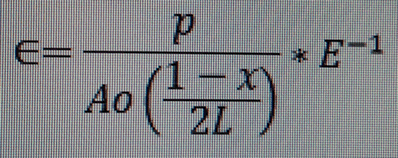# Integration issue

3 posts / 0 new
vipa2000
Integration issue

After deriving an equation I have ended up with equation where I have a complex denominator and I am not sure how to simplify. I am trying to work out as a function of x. Equation link is below. Thanks in advance.

https://www.flickr.com/photos/baldypaul/32722700004/in/datetaken/

Jhun Vert\begin{align} \dfrac{p}{\dfrac{Ao\left( \dfrac{1 - x}{2L} \right)}{E}} & = \dfrac{2pEL}{Ao(1 - x)} \\ & = \dfrac{2pEL}{Ao} \cdot \dfrac{1}{1 - x} \end{align}

You posted this with a title "integration issue" under calculus, which I presume you wanted to do this:
$\displaystyle \dfrac{2pEL}{Ao} \int \dfrac{dx}{1 - x} = -\dfrac{2pEL}{Ao}\ln (1 - x) + C$

Side Note:

Allow me to bring up your previous post which is a little similar to the equation in this post.The only difference is E.

Note that
$\dfrac{p}{\dfrac{Ao\left( \dfrac{1 - x}{2L} \right)}{E}} = \dfrac{p}{Ao\left( \dfrac{1 - x}{2L} \right)} * E$

while
$\dfrac{p}{Ao\left( \dfrac{1 - x}{2L} \right)} * E^{-1} = \dfrac{p}{AoE\left( \dfrac{1 - x}{2L} \right)}$

I am not sure though if this side note is still relevant to you.

vipa2000

Romel, this is excellent.

If you get chance, could you explain some of the steps i.e. not sure how E is positive and a numerator, and in addition the ln(1-x). Thanks in advance Paul

Subscribe to MATHalino on